## Specifications

## Specifications

Japan

### Three-phase, 200V series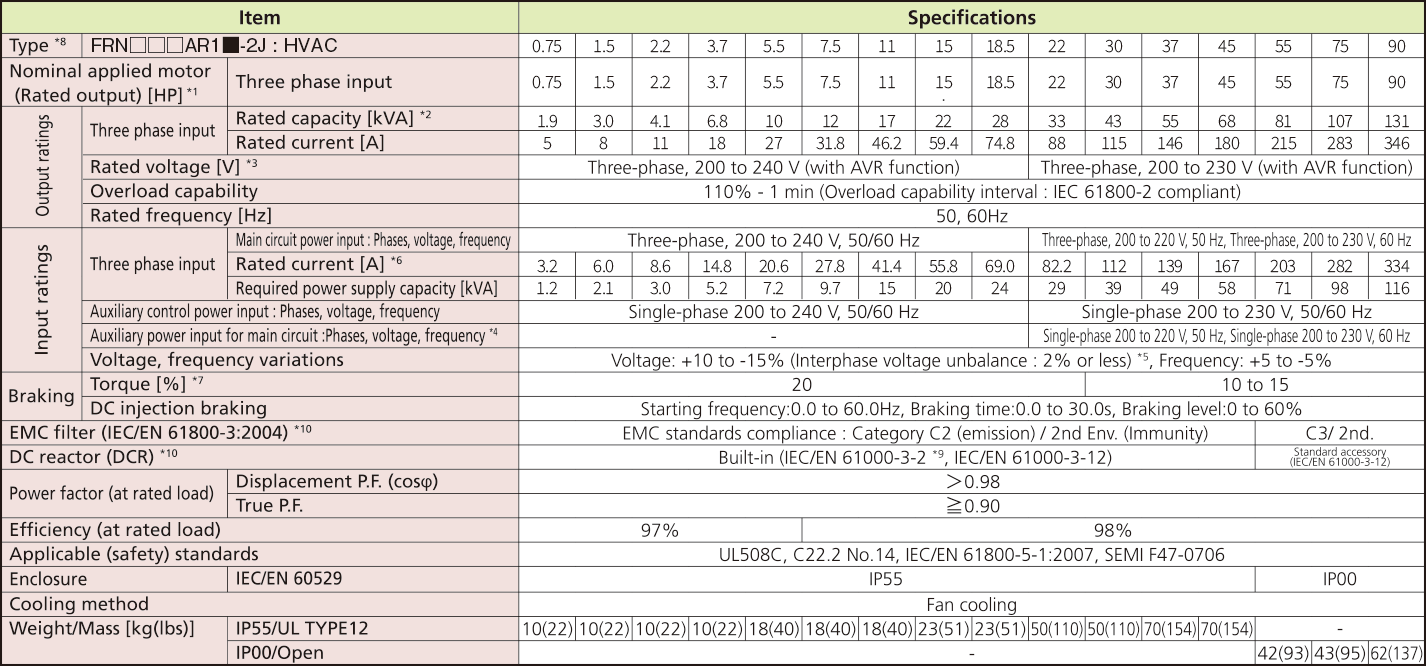### 3-phase, 400V series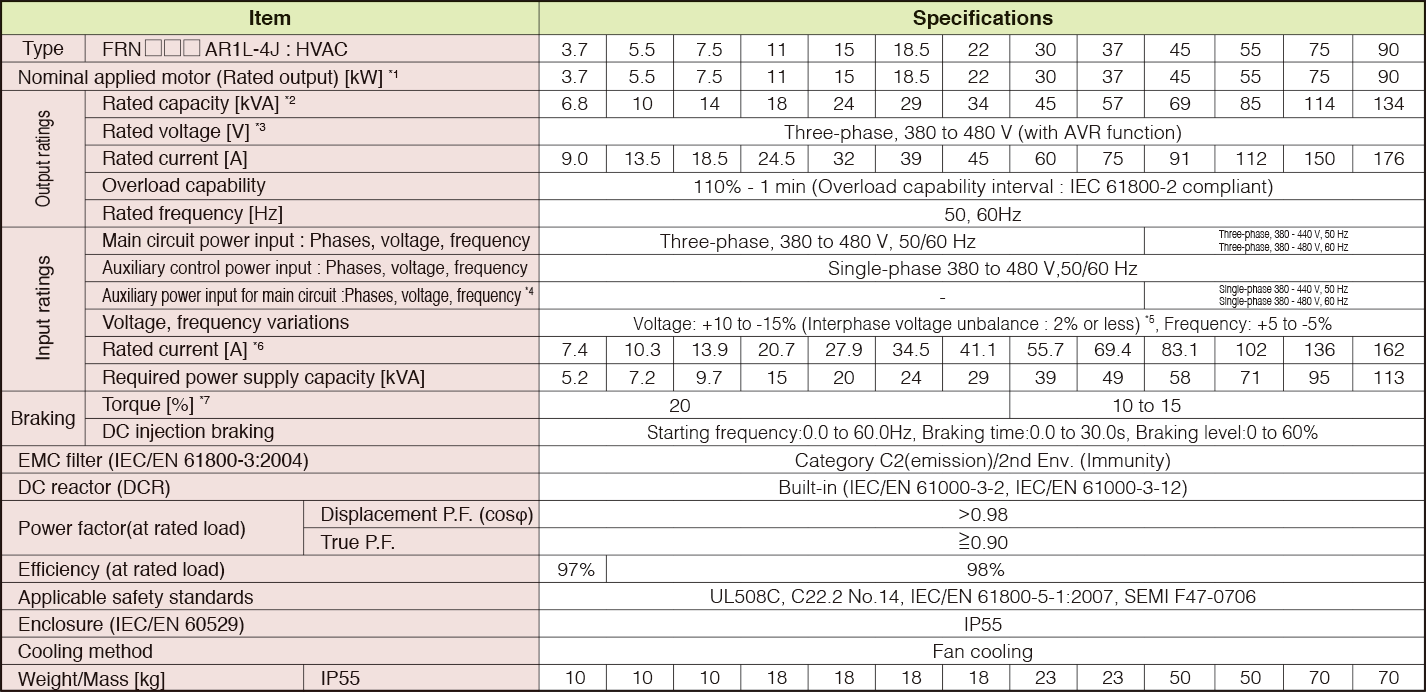*1)
Fuji 4-pole standard motor.
*2)
Rated capacity is calculated by assuming the output rated voltage as 440 V.
*3)
Output voltage cannot exceed the power supply voltage.
*4)
The auxiliary power input is used as an AC power input when combining the unit to DC power supply such as high power factor PWM converter with power regenerative function. (Generally not to be used.)
*5)
Voltage unbalance [%] = (Max. voltage [V] - Min. voltage [V])/Three-phase average voltage [V] x 67 (See IEC61800-3.) If this value is 2 to 3%, use an optional AC reactor (ACR).
*6)
The value is calculated on assumption that the inverter is connected with a power supply 400V, 50Hz and Rsce=120.
*7)
Average braking torque for the motor running alone. (It varies with the efficiency of the motor.)
Europe

### 3-phase, 400V series (0.75 to 55kW)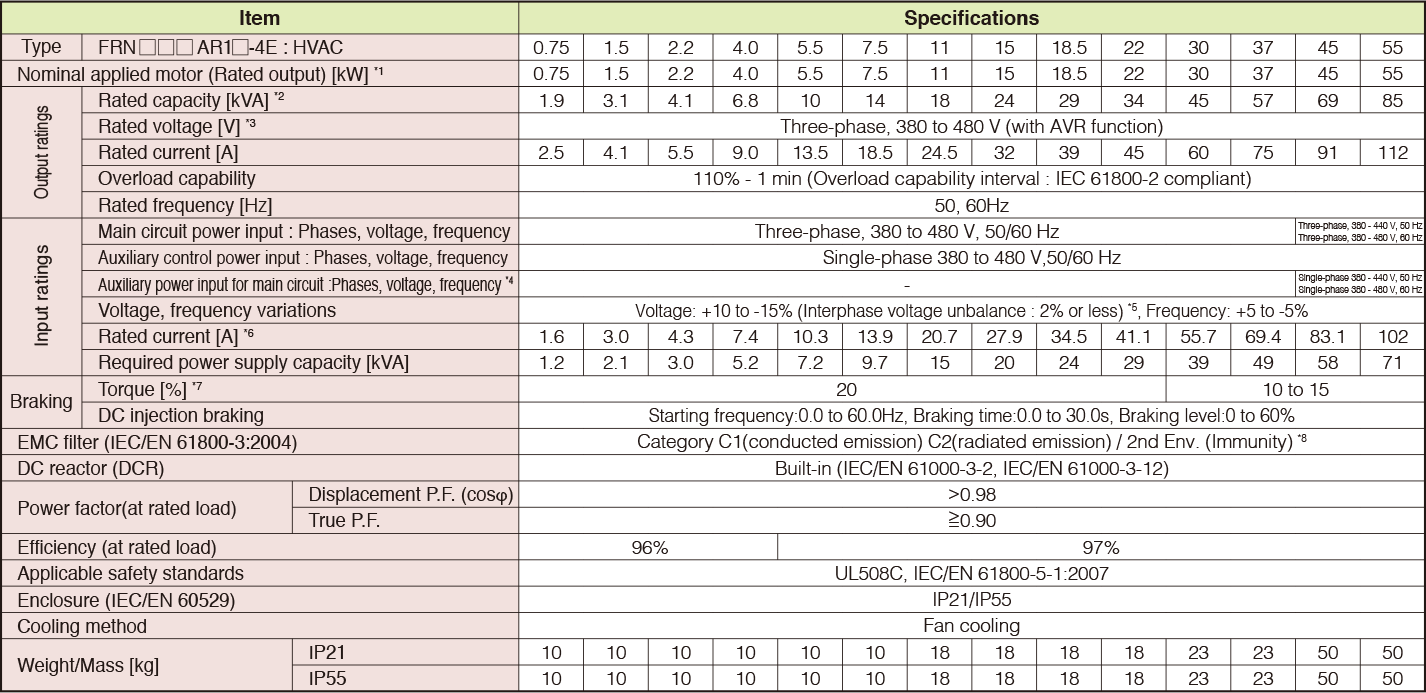### 3-phase, 400V series (75 to 710kW)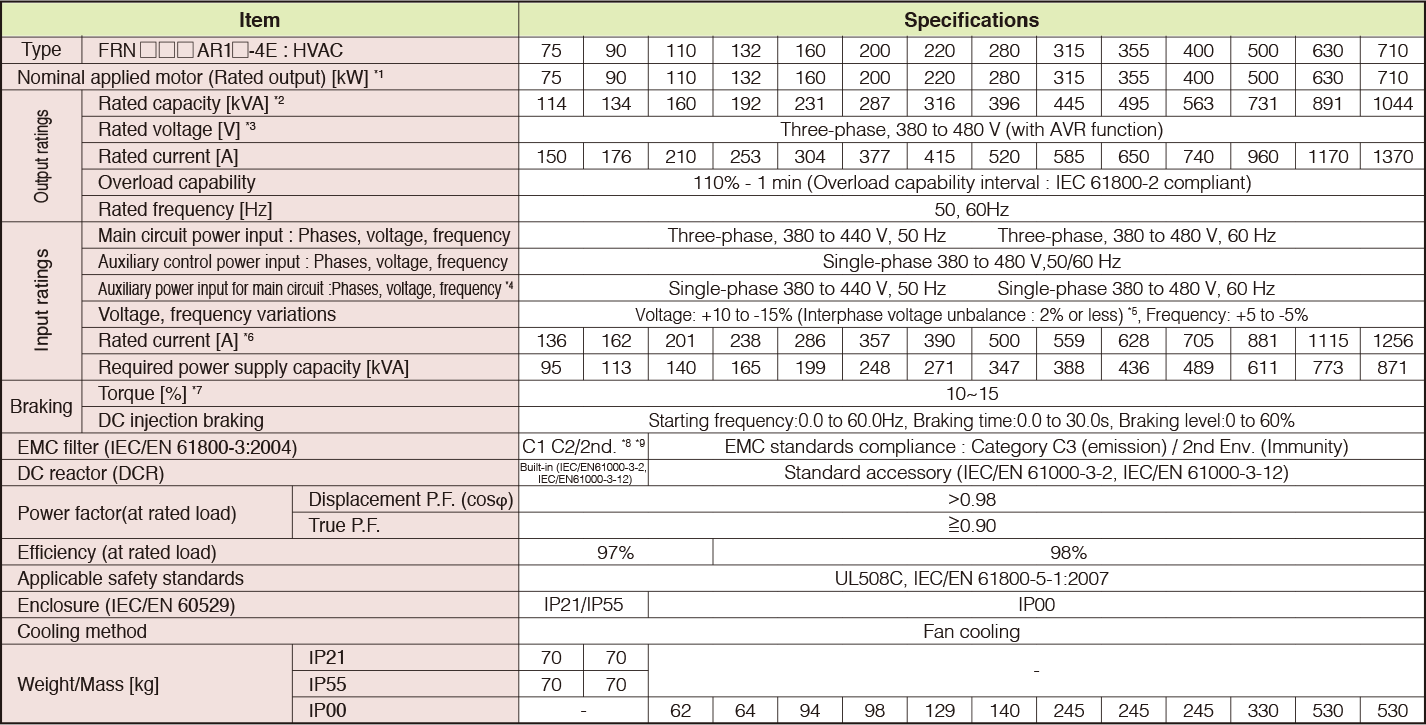*1)
Fuji 4-pole standard motor.
*2)
Rated capacity is calculated by assuming the output rated voltage as 440 V.
*3)
Output voltage cannot exceed the power supply voltage.
*4)
The auxiliary power input is used as an AC power input when combining the unit to DC power supply such as high power factor PWM converter with power regenerative function. (Generally not to be used.)
*5)
Voltage unbalance [%] = (Max. voltage [V] - Min. voltage [V])/Three-phase average voltage [V] x 67 (See IEC61800-3.) If this value is 2 to 3%, use an optional AC reactor (ACR).
*6)
The value is calculated on assumption that the inverter is connected with a power supply 400V, 50Hz and Rsce=120.
*7)
Average braking torque for the motor running alone. (It varies with the efficiency of the motor.)
*8)
To provide compatibility for EMC category C1, ensure a motor power cable leng h of 10 m or less, a carrier frequency of 4kHz or less, and ground the E1 and E2 terminals.
*9)
Category C1(conducted emission) C2(radiated emission) / 2nd Env. (Immunity)
North America

### Three-phase 230 V Series (1 to 125 HP)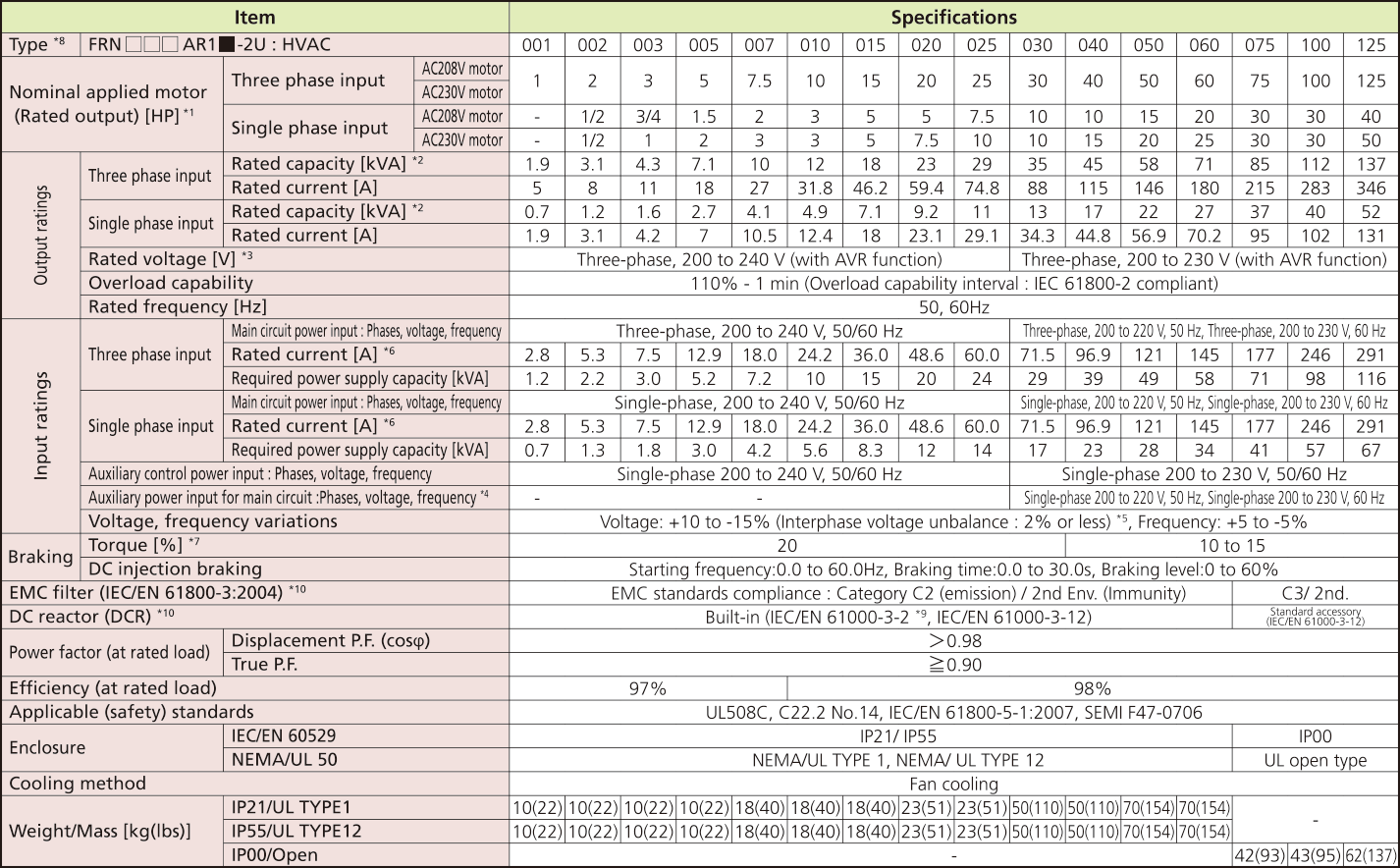*1)
US 4-pole standard induction motor.
*2)
Rated capacity is calculated by assuming the output rated voltage as 230 V.
*3)
Output voltage cannot exceed the power supply voltage. At single-phase input use, the output voltage may be lower than three-phase input.
*4)
The auxiliary power input is used as an AC power input when combining the unit to DC power supply such as high power factor PWM converter with power regenerative function. (Generally not to be used.)
*5)
Voltage unbalance [%] = (Max. voltage [V] - Min. voltage [V])/Three-phase average voltage [V] x 67 (See IEC61800-3.)If this value is 2 to 3%, use an optional AC reactor (ACR).
*6)
The value is calculated on assumption that the inverter is connected with a power supply 230V, 50Hz and Rsce=120.
*7)
Average braking torque for the motor running alone. (It varies with the efficiency of the motor.)
*8)
The box (□) replaces an numeric letter depending on the drive capacity.
The box (■) replaces an alphabetic letter depending on the enclosure. M (IP21/UL TYPE1), L (IP55/UL TYPE12) or S (IP00/Open)
*9)
It is applicable when the power supply is supplied from 3-phase 200V series transformer which is through 3-phase 400V series transformer.
*10)
EMC filters and DCR does not conform to each corresponding standards when single phase input use.

### Three-phase 460 V Series (1 to 75 HP)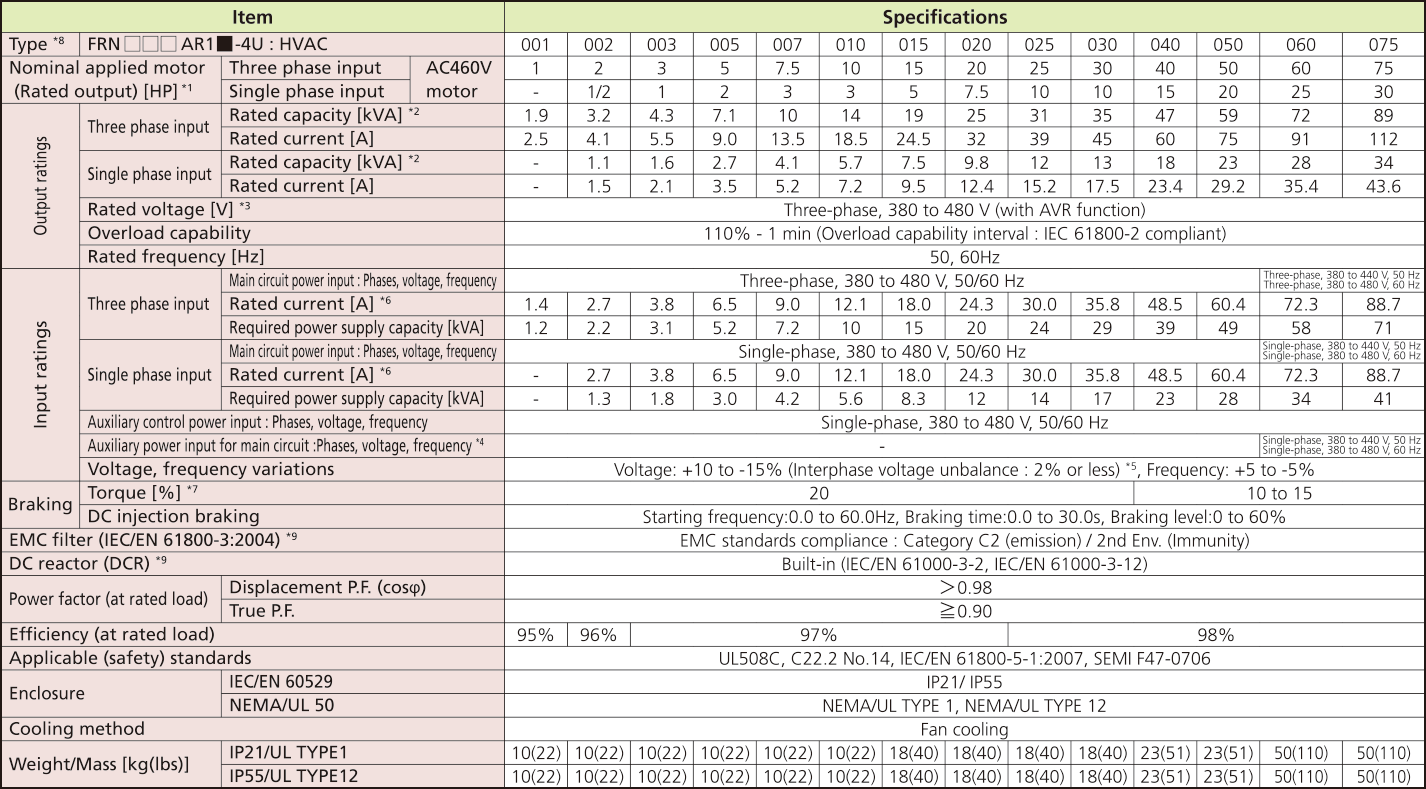### Three-phase 460 V Series (100 to 1000 HP)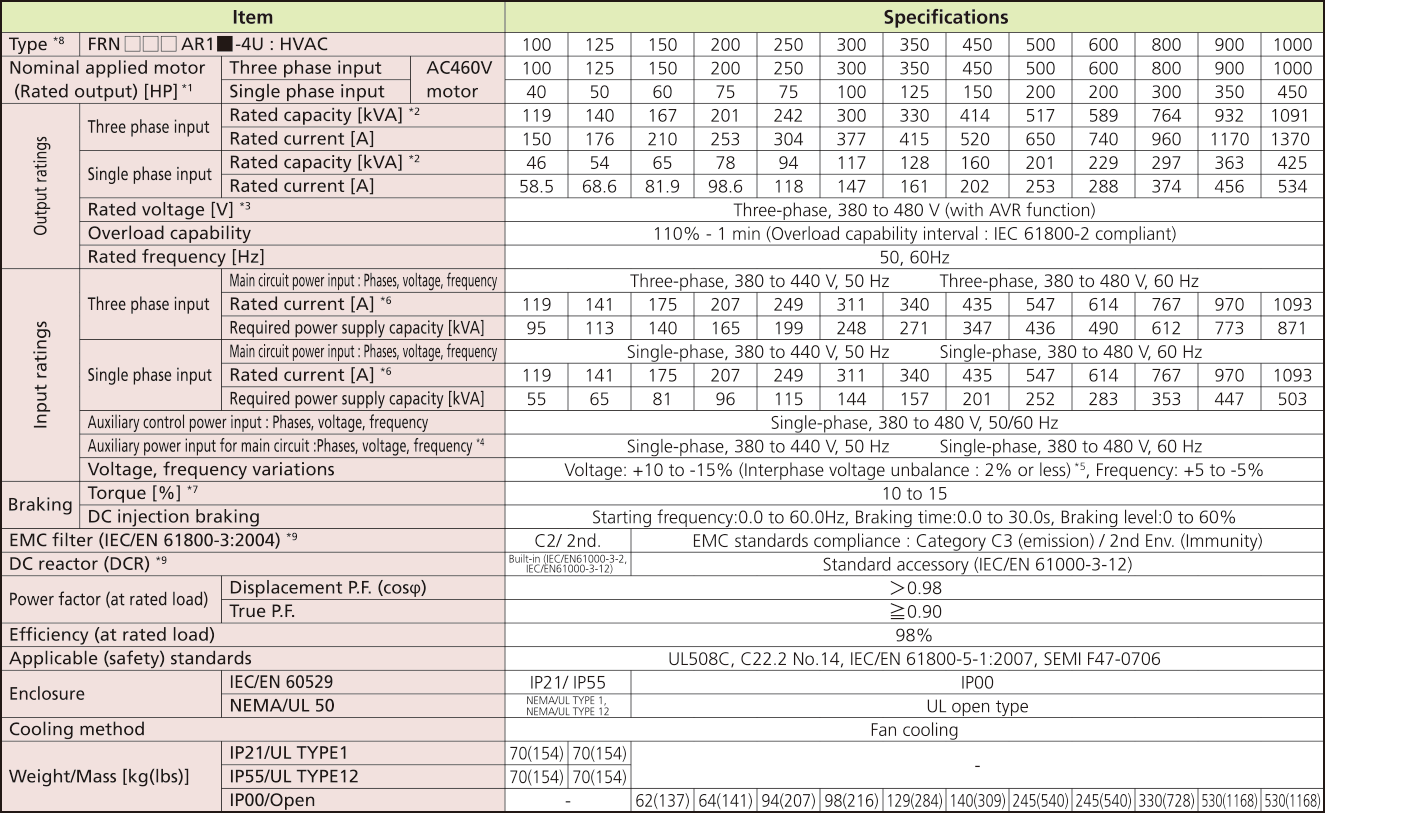*1)
US 4-pole standard induction motor.
*2)
Rated capacity is calculated by assuming the output rated voltage as 460 V.
*3)
Output voltage cannot exceed the power supply voltage. At single-phase input use, the output voltage may be lower than three-phase input.
*4)
The auxiliary power input is used as an AC power input when combining the unit to DC power supply such as high power factor PWM converter with power regenerative function. (Generally not to be used.)
*5)
Voltage unbalance [%] = (Max. voltage [V] - Min. voltage [V])/Three-phase average voltage [V] x 67 (See IEC61800-3.) If this value is 2 to 3%, use an optional AC reactor (ACR).
*6)
The value is calculated on assumption that the inverter is connected with a power supply 460V, 50Hz and Rsce=120.
*7)
Average braking torque for the motor running alone. (It varies with the efficiency of the motor.)
*8)
The box (□) replaces an numeric letter depending on the drive capacity. The box (■) replaces an alphabetic letter depending on the enclosure. M (IP21/UL TYPE1), L (IP55/UL TYPE12) or S (IP00/Open)
*9)
EMC filters and DCR does not conform to each corresponding standards when single phase input use.

### Three-phase 575 V Series (1 to 30 HP)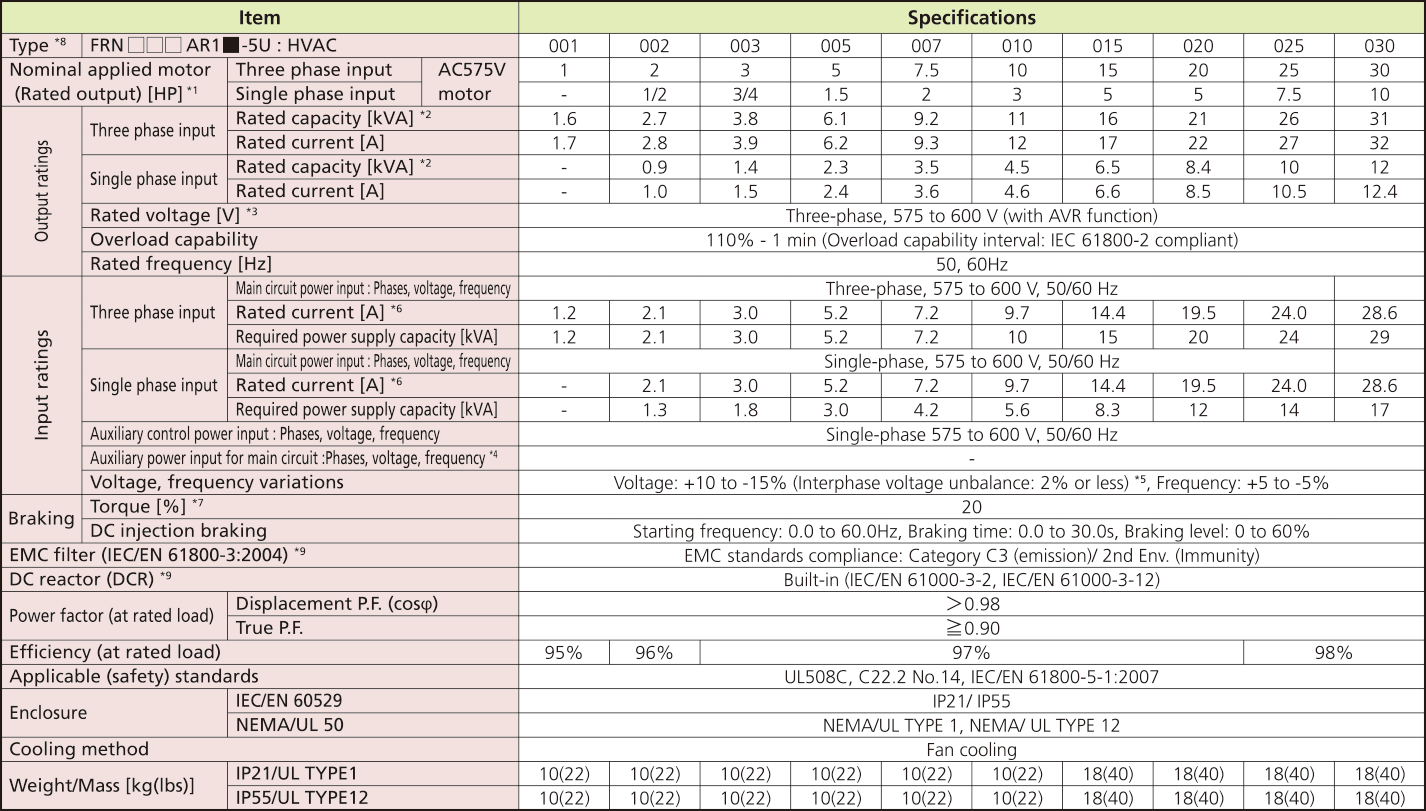### Three-phase 575 V Series (40 to 300 HP)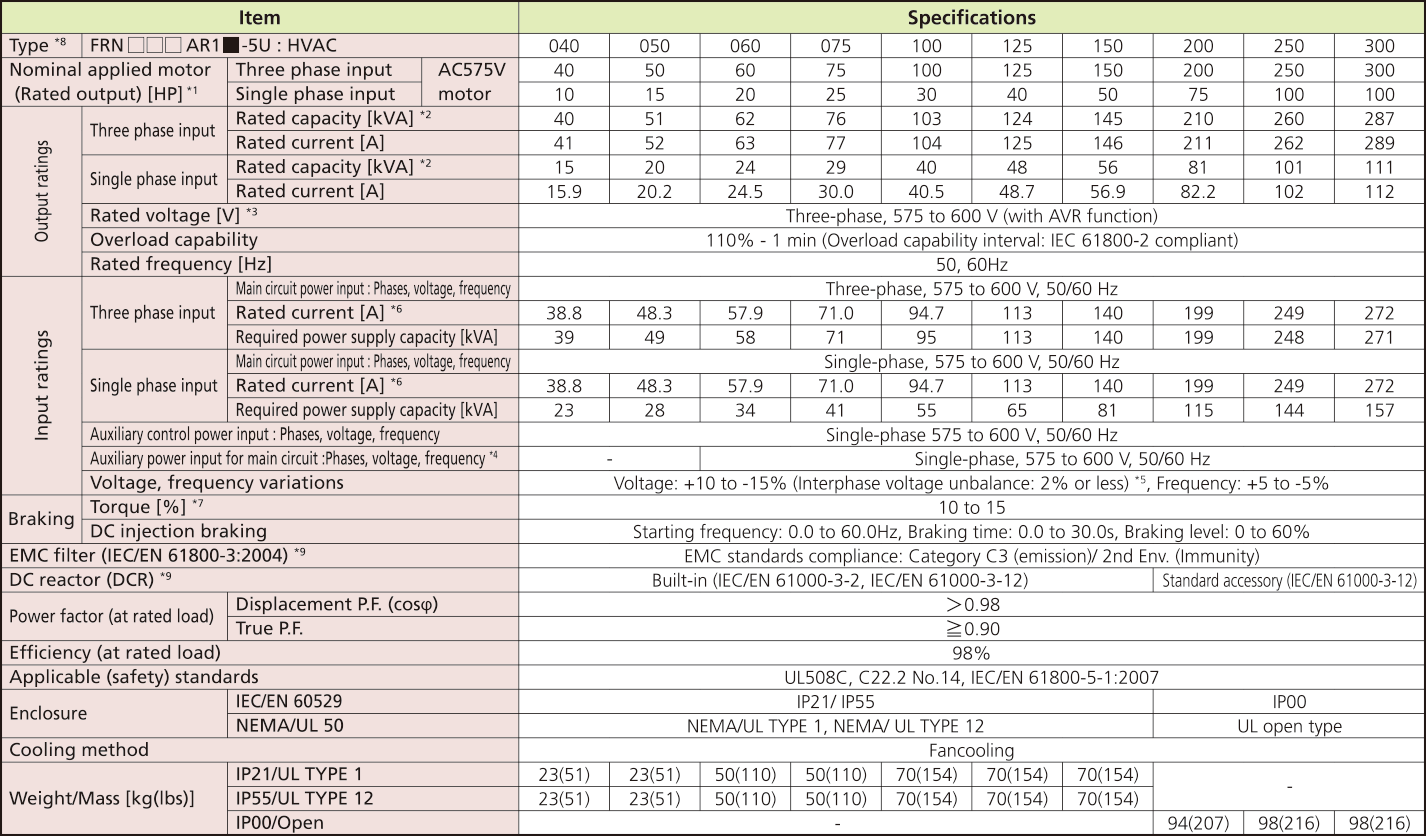*1)
US 4-pole standard induction motor.
*2)
Rated capacity is calculated by assuming the output rated voltage as 575 V.
*3)
Output voltage cannot exceed the power supply voltage. At single-phase input use, the output voltage may be lower than three-phase input.
*4)
The auxiliary power input is used as an AC power input when combining the unit to DC power supply such as high power factor PWM converter with power regenerative function. (Generally not to be used.)
*5)
Voltage unbalance [%] = (Max. voltage [V] - Min. voltage [V])/Three-phase average voltage [V] x 67 (See IEC61800-3.) If this value is 2 to 3%, use an optional AC reactor (ACR).
*6)
The value is calculated on assumption that the inverter is connected with a power supply 575V, 50Hz and Rsce=120.
*7)
Average braking torque for the motor running alone. (It varies with the efficiency of the motor.)
*8)
The underline (□) replaces an numeric letter depending on the drive capacity. The box (■) replaces an alphabetic letter depending on the enclosure. M (IP21), L (IP55) or S (IP00)
*9)
EMC filters and DCR does not conform to each corresponding standards when single phase input use.
Asia

### 3-phase, 400V series (0.75 to 55kW)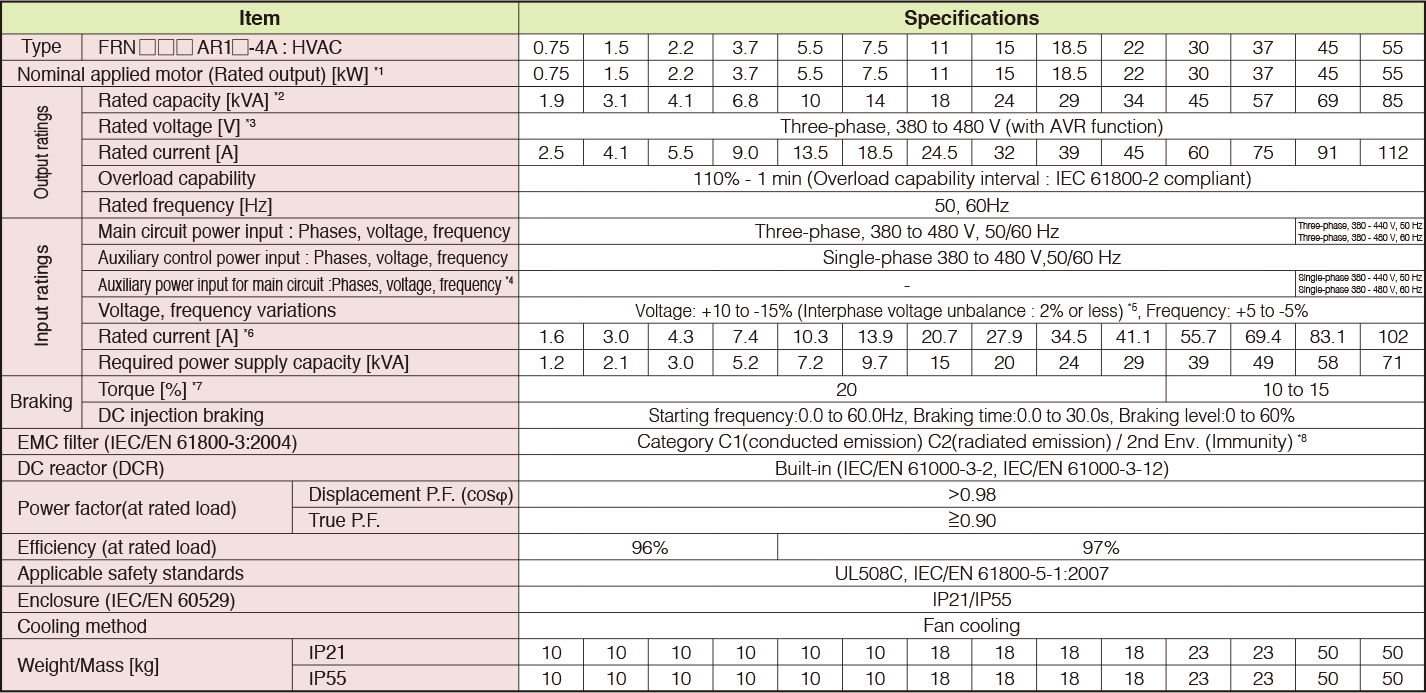### 3-phase, 400V series (75 to 710kW)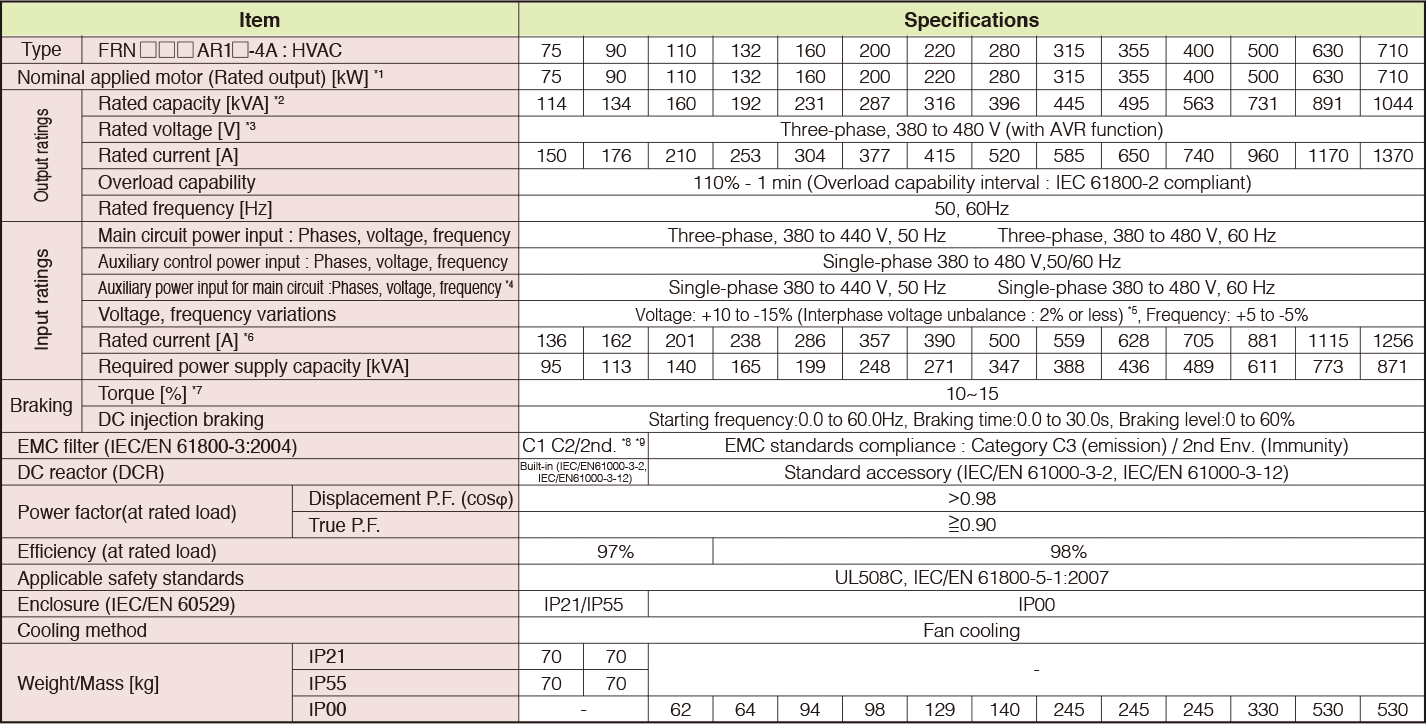*1)
Fuji 4-pole standard motor.
*2)
Rated capacity is calculated by assuming the output rated voltage as 440 V.
*3)
Output voltage cannot exceed the power supply voltage.
*4)
The auxiliary power input is used as an AC power input when combining the unit to DC power supply such as high power factor PWM converter with power regenerative function. (Generally not to be used.)
*5)
Voltage unbalance [%] = (Max. voltage [V] - Min. voltage [V])/Three-phase average voltage [V] x 67 (See IEC61800-3.) If this value is 2 to 3%, use an optional AC reactor (ACR).
*6)
The value is calculated on assumption that the inverter is connected with a power supply 400V, 50Hz and Rsce=120.
*7)
Average braking torque for the motor running alone. (It varies with the efficiency of the motor.)
*8)
To provide compatibility for EMC category C1, ensure a motor power cable leng h of 10 m or less, a carrier frequency of 4kHz or less, and ground the E1 and E2 terminals.
*9)
Category C1(conducted emission) C2(radiated emission) / 2nd Env. (Immunity)